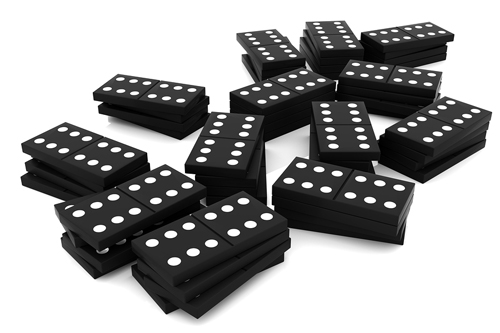# Domino domination!Let $f(n)$ be the number of ways in which one can cover a $3 \times n$ rectangle with dominoes (rectangles with side length $1 \times 2$). Find the sum of the digits of $f(100)$.

Explicit examples

• $f(3)=0$

• $f(4)=11$

• $f(10)=571$

×